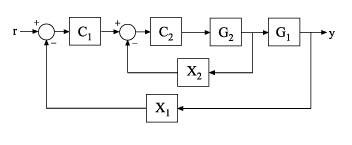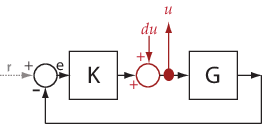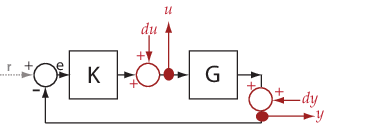# getSensitivity

Sensitivity function from generalized model of control system

## Syntax

``S = getSensitivity(T,location)``
``S = getSensitivity(T,location,opening)``

## Description

example

````S = getSensitivity(T,location)` returns the sensitivity function at the specified location for a generalized model of a control system.```

example

````S = getSensitivity(T,location,opening)` specifies additional loop openings for the sensitivity function calculation. Use an opening, for example, to calculate the sensitivity function of an inner loop, with the outer loop open.If `opening` and `location` list the same point, the software opens the loop after measuring the signal at the point.```

## Examples

collapse all

Compute the sensitivity at the plant input, marked by the analysis point `X`.Create a model of the system by specifying and connecting a numeric LTI plant model `G`, a tunable controller `C`, and the `AnalysisPoint` block `X`. Use the `AnalysisPoint` block to mark the location where you assess the sensitivity (plant input in this example).

```G = tf(,[1 5]); C = tunablePID('C','p'); C.Kp.Value = 3; X = AnalysisPoint('X'); T = feedback(G*X*C,1);```

`T` is a `genss` model that represents the closed-loop response of the control system from $r$ to $y$. The model contains the `AnalysisPoint` block, `X`, that identifies the analysis point.

Calculate the sensitivity, $S$, at `X`.

```S = getSensitivity(T,'X'); tf(S)```
```ans = From input "X" to output "X": s + 5 ----- s + 8 Continuous-time transfer function. ```

Calculate the inner-loop sensitivity at the output of `G2`, with the outer loop open.Create a model of the system by specifying and connecting the numeric plant models, tunable controllers, and `AnalysisPoint` blocks. `G1` and `G2` are plant models, `C1` and `C2` are tunable controllers, and `X1` and `X2` are `AnalysisPoint` blocks that mark potential loop-opening locations.

```G1 = tf(10,[1 10]); G2 = tf([1 2],[1 0.2 10]); C1 = tunablePID('C','pi'); C2 = tunableGain('G',1); X1 = AnalysisPoint('X1'); X2 = AnalysisPoint('X2'); T = feedback(G1*feedback(G2*C2,X2)*C1,X1);```

Calculate the sensitivity, $S$, at `X2`, with the outer loop open at `X1`.

```S = getSensitivity(T,'X2','X1'); tf(S)```
```ans = From input "X2" to output "X2": s^2 + 0.2 s + 10 ---------------- s^2 + 1.2 s + 12 Continuous-time transfer function. ```

## Input Arguments

collapse all

Model of a control system, specified as a generalized state-space model (`genss`).

Locations at which you can perform sensitivity analysis or open loops are marked by `AnalysisPoint` blocks in `T`. Use `getPoints(T)` to get the list of such locations.

Location at which you calculate the sensitivity function, specified as a character vector or cell array of character vectors. To extract the sensitivity function at multiple locations, use a cell array of character vectors.

Each specified location must match an analysis point in `T`. Analysis points are marked using `AnalysisPoint` blocks. To get the list of available analysis points in `T`, use `getPoints(T)`.

Example: `'u'` or `{'u','y'}`

Additional loop opening used to calculate the sensitivity function, specified as a character vector or cell array of character vectors. To open the loop at multiple locations, use a cell array of character vectors.

Each specified opening must match an analysis point in `T`. Analysis points are marked using `AnalysisPoint` blocks. To get the list of available analysis points in `T`, use `getPoints(T)`.

Use an opening, for example, to calculate the sensitivity function of an inner loop, with the outer loop open.

If `opening` and `location` list the same point, the software opens the loop after measuring the signal at the point.

Example: `'y_outer'` or `{'y_outer','y_outer2'}`

## Output Arguments

collapse all

Sensitivity function of the control system, `T`, measured at `location`, returned as a generalized state-space model (`genss`).

• If `location` specifies a single analysis point, then `S` is a SISO `genss` model.

• If `location` is a vector signal, or specifies multiple analysis points, then `S` is a MIMO `genss` model.

collapse all

### Sensitivity Function

The sensitivity function, also referred to simply as sensitivity, measures how sensitive a signal is to an added disturbance. Feedback reduces the sensitivity in the frequency band where the open-loop gain is greater than `1`.

Consider the following model:The sensitivity, Su, at `u` is defined as the transfer function from `du` to `u`:`$\begin{array}{l}u=du-KGu\\ \to \left(I+KG\right)u=du\\ \to u=\underset{{S}_{u}}{\underbrace{{\left(I+KG\right)}^{-1}}}du.\end{array}$`

Here, I is an identity matrix of the same size as KG.

Sensitivity at multiple locations, for example, `u` and `y`, is defined as the MIMO transfer function from the disturbances to sensitivity measurements:`$S=\left[\begin{array}{cc}{S}_{du\to u}& {S}_{dy\to u}\\ {S}_{du\to y}& {S}_{dy\to y}\end{array}\right].$`

## Version History

Introduced in R2014a